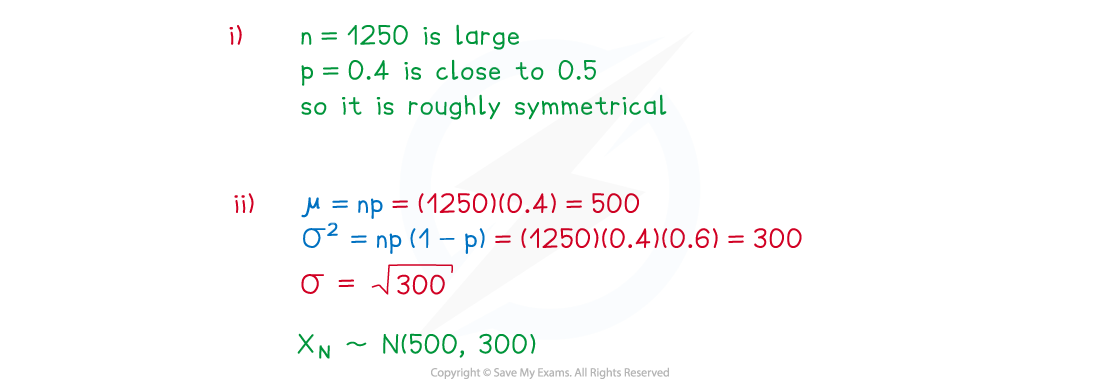# AQA A Level Maths: Statistics复习笔记4.4.2 Normal Approximation of Binomial

### Normal Approximation of Binomial

#### Worked Example(i)Explain why a normal distribution can be used to approximate the distribution of .
(ii)Write down the approximating normal distribution.#### Exam Tip

• In the exam, you will only be asked to describe the approximating distribution and not to use it.